#### Chapter 16 Circles R.D. Sharma Solutions for Class 9th MCQ's

Multiple Choice Questions

1. If the length of a chord of a circle is 16 cm and is at a distance of 15 cm from the centre of the circle, then the radius of the circle is
(a) 15 cm
(b) 16 cm
(c) 17 cm
(d) 34 cm

Solution2. The radius of a circle is 6 cm. The perpendicular distance from the centre of the circle to the chord which is 8 cm in length, is
(a) √5 cm
(b) 2√5 cm
(c) 2√7 cm
(d) √7 cm

Solution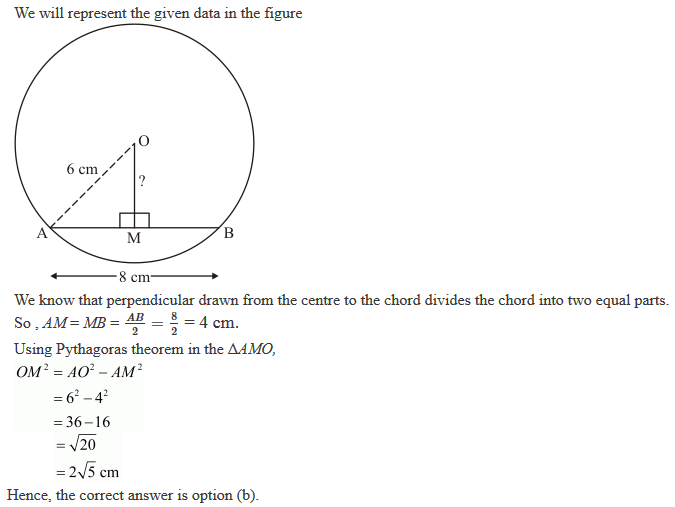3. If O is the centre of a circle of radius r and AB is a chord of the circle at a distance r/2 from O, then ∠BAO =
(a) 60°
(b) 45°
(c) 30°
(d) 15°

Solution4. ABCD is a cyclic quadrilateral such that ∠ADB = 30° and ∠DCA = 80°, then ∠DAB =
(a) 70°
(b) 100°
(c) 125°
(d) 150°

Solution5. A chord of length 14 cm is at a distance of 6 cm from the centre of a circle. The length of another chord at a distance of 2 cm from the centre of the circle is
(a) 12 cm
(b) 14 cm
(c) 16 cm
(d) 18 cm

Solution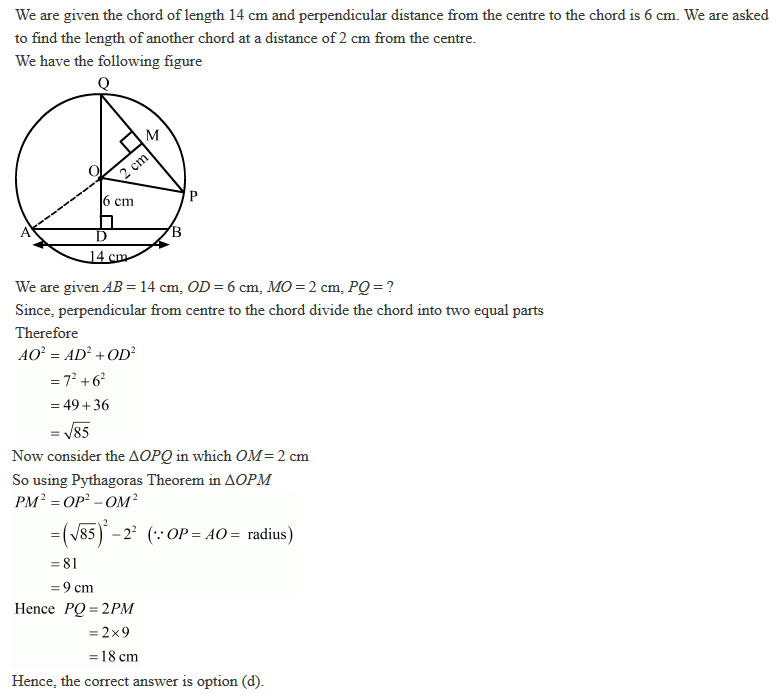6. One chord of a circle is known to be 10 cm. The radius of this circle must be
(a) 5 cm
(b) greater than 5 cm
(c) greater than or equal to 5 cm
(d) less than 5 cm

Solution

(b) greater than 5 cm
We are given length of a chord to be 10 cm and we have to give information about the radius of the circle.
Since in any circle, diameter of the circle is greater then any chord.
So diameter > 10
⇒ 2r > 10
⇒ r > 5 cm Hence, the correct answer is option (b)

7. ABC is a triangle with B as right angle, AC = 5 cm and AB = 4 cm. A circle is drawn with A as centre and AC as radius. The length of the chord of this circle passing through C and B is
(a) 3 cm
(b) 4 cm
(c) 5 cm
(d) 6 cm

Solution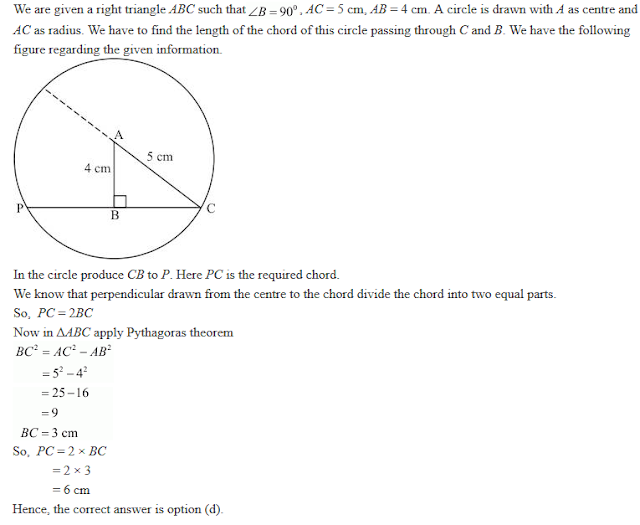8. If AB, BC and CD are equal chords of a circle with O as centre and AD diameter, than ∠AOB =
(a) 60°
(b) 90°
(c) 120°
(d) none of these

Solution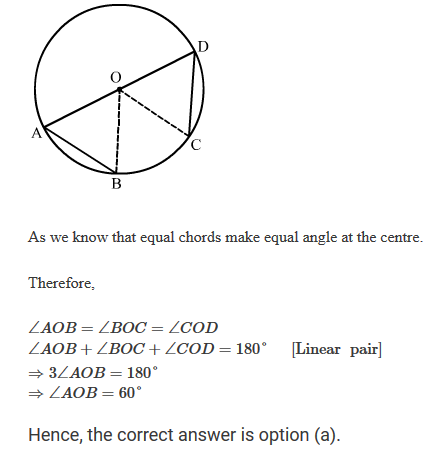9. Let C be the mid-point of an arc AB of a circle such that mAB = 183°. If the region bounded by the arc ACB and the line segment AB is denoted by S, then the centre O of the circle lies:
(a) in the interior of S
(b) in the exterior of S
(c) on the segment AB
(d) on AB and bisects AB

Solution10. In a circle, the major arc is 3 times the minor arc. The corresponding central angles and the degree measures of two arcs are
(a) 90° and 270°
(b) 90° and 90°
(c) 270° and 90°
(d) 60° and 210°

Solution11. If A and B are two points on a circle such that m(AB) = 260°. A possible value for the angle subtended by arc BA at a point on the circle is:
(a) 100°
(b) 75°
(c) 50°
(d) 25°

Solution19. An equilateral triangle ABC is inscribed in a circle with centre O. The measures of ∠BOC is
(a) 30°
(b) 60°
(c) 90°
(d) 120°

Solution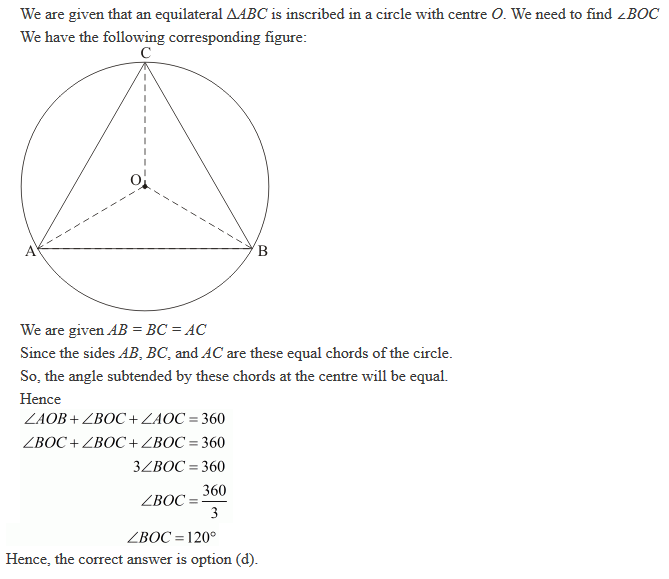13. In a circle with centre O, AB and CD are two diameters perpendicular to each other. The length of chord AC is
(a) 2AB
(b) √2
(c) 1/2 AB
(d) 1/√2 AB

Solution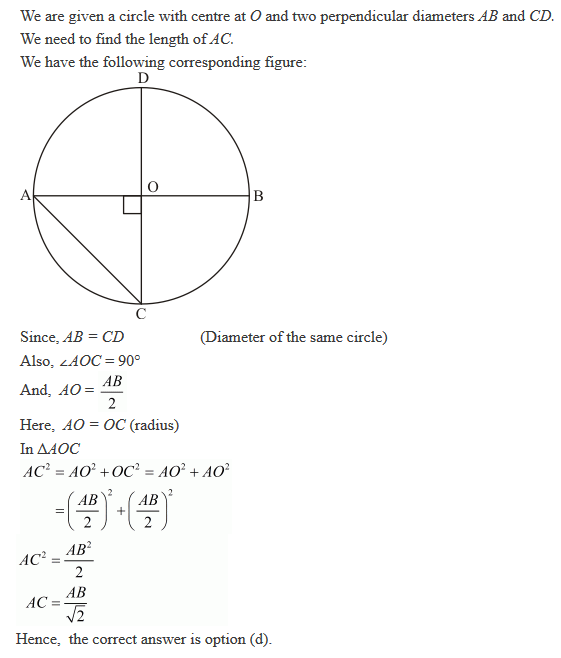14.Two equal circles of radius r intersect such that each passes through the centre of the other. The length of the common chord of the circle is
(a) √r
(b) √2r AB
(c) √3r
(d) √3/2

Solution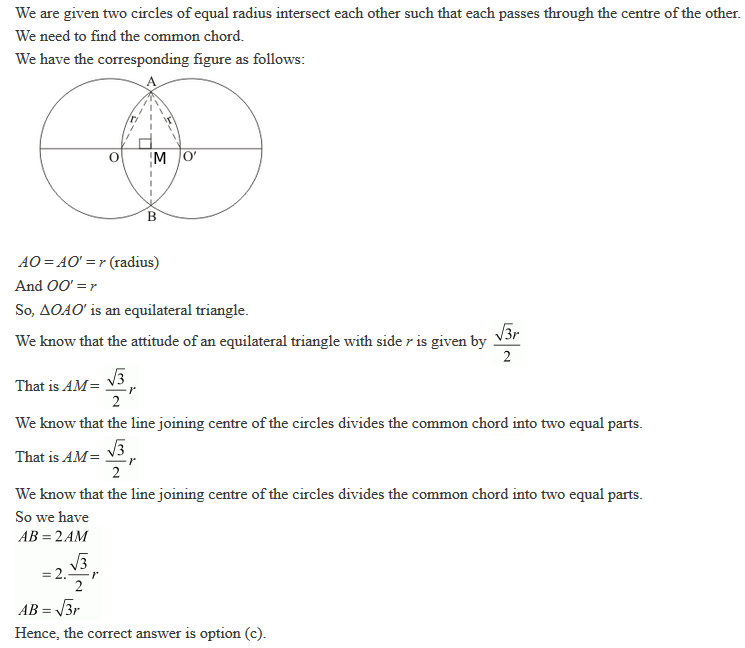15. If AB is a chord of a circle, P and Q are the two points on the circle different from A and B, then
(a) ∠APB = ∠AQB
(b) ∠APB + ∠AQB = 180° or ∠APB = ∠AQB
(c) ∠APB + ∠AQB = 90°
(d) ∠APB + ∠AQB = 180°

Solution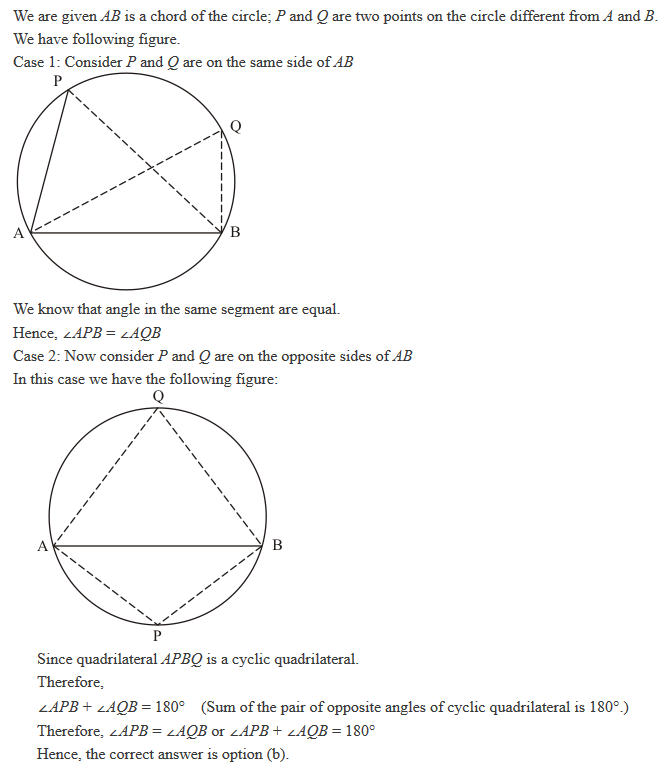16. If two diameters of a circle intersect each other at right angles, then quadrilateral formed by joining their end points is a
(a) rhombus
(b) rectangle
(c) parallelogram
(d) square

Solution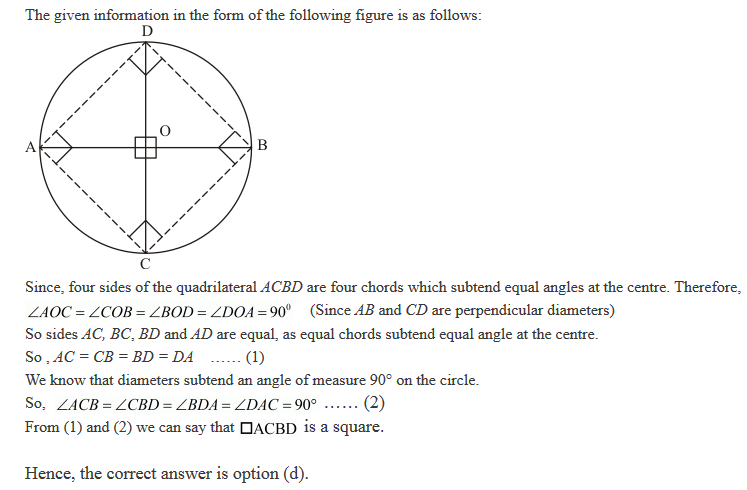17. If ABC is an arc of a circle and ∠ABC = 135°, then the ratio of arc ABC to the circumference is:
(a) 1:4
(b) 3:4
(c) 3:8
(d) 1:2

Solution

The length of an arc subtending an angle '𝜃' in a circle of radius 'r' is given by the formula, Length of the arc = 2πr(𝜃/360°)
Here, it is given that the arc subtends an angle of 135° with its centre. So, the length of the given arc in a circle with radius 'r' given as
Length of the arc = 2πr(135°/360°)
The circumference of the same circle with radius 'r' is given as 2πr
The ratio between the lengths of the arc and the circumference of the circle will be,
Length of the arc/Circumference of the circle = 135°(2πr)/360°(2πr)
= 135°/360° = 3/8
Hence, the correct answer is option (c).
18. The chord of a circle is equal to its radius. The angle subtended by this chord at the minor arc of the circle is
(a) 60°
(b) 75°
(c) 120°
(d) 150°

Solution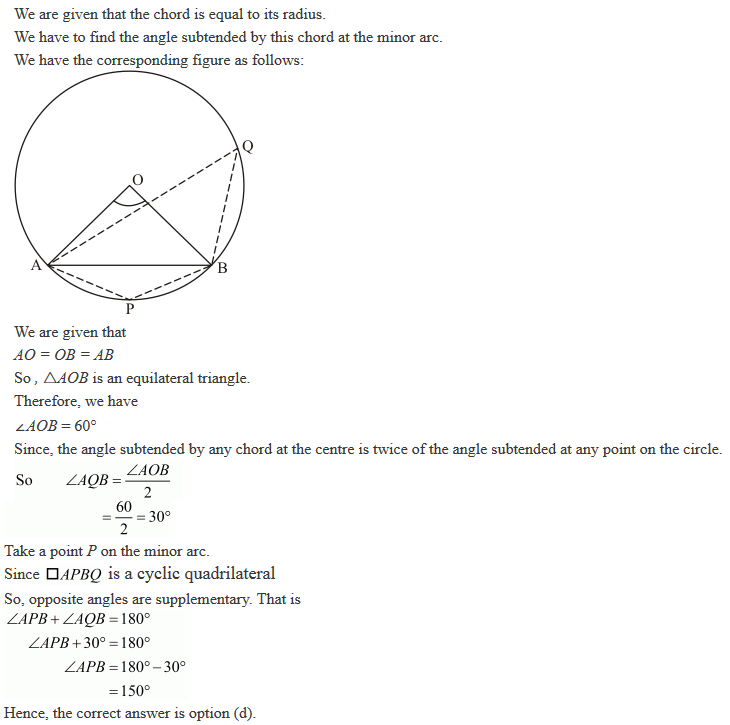19. PQRS is a cyclic quadrilateral such that PR is a diameter of the circle. If ∠QPR = 67° and ∠SPR = 72°, then ∠QRS =
(a) 41°
(b) 23°
(c) 67°
(d) 18°

Solution20. If A , B, C are three points on a circle with centre O such that ∠AOB = 90° and ∠BOC = 120°, then ∠ABC =
(a) 60°
(b) 75°
(c) 90°
(d) 135°

Solution21. AB and CD are two parallel chords of a circle with centre O such that AB = 6 cm and CD = 12 cm. The chords are on the same side of the centre and the distance between them is 3 cm. The radius of the circle is
(a) 6 cm
(b) 5√2 cm
(c) 7 cm
(d) 3√5 cm

Solution22. In a circle of radius 17 cm, two parallel chords are drawn on opposite side of a diameter. The distance between the chords is 23 cm. If the length of one chord is 16 cm, then the length of the other.
(a) 34 cm
(b) 15 cm
(c) 23 cm
(d) 30 cm

Solution23. The greatest chord of a circle is called its
(b) secant
(c) diameter
(d) none of these

Solution

(c) diameter
The greatest chord in a circle is the diameter of the circle.
Hence the correct answer is option (c).

24. Angle formed in minor segment of a circle is
(a) acute
(b) obtuse
(c) right angle
(d) none of these

Solution

Whenever a chord is drawn in a circle two segments are formed. One is called the minor segment while the other is called the major segment. The angle formed by the chord in the minor segment will always be obtuse. Hence the correct answer is option (b) .

25. Number of circles that can be drawn through three non-collinear points is
(a) 1
(b) 0
(c) 2
(d) 3

Solution26. In Fig. 16.201, O is the centre of the circle such that ∠AOC = 130°, then ∠ABC =
(a) 130°
(b) 115°
(c) 65°
(d) 165°Solution27. In Fig. 16.202, if chords AB and CD of the circle intersect each other at right angles, then x + y =
(a) 45°
(b) 60°
(c) 75°
(d) 90°Solution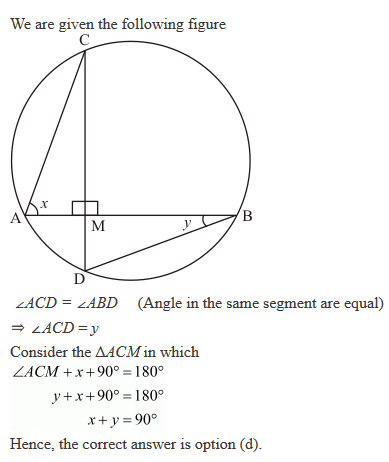28. In Fig. 16.203, if ∠ABC = 45°, then ∠AOC =
(a) 45°
(b) 60°
(c) 75°
(d) 90°Solution29. In Fig. 16.204, chords AD and BC intersect each other at right angles at a point P. If ∠DAB = 35°, then
(a) 35°
(b) 45°
(c) 55°
(d) 65°Solution30. In Fig. 16.205, O is the centre of the circle and ∠BDC = 42°. The measure of ∠ACB is

(a) 42°
(b) 48°
(c) 58°
(d) 52°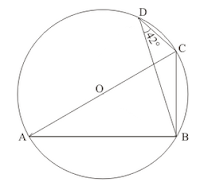Solution